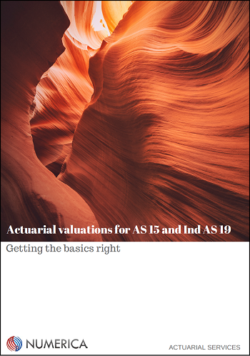The concept of actuarial gain or loss is central to any actuarial valuation, but is widely misunderstood. A clear understanding of this concept could pre-empt a range of questions and free up time and resources tied up in the actuarial valuation process.

An understanding of actuarial loss under AS 15, or ‘remeasurement’ under Ind AS 19, can be considered the holy grail of an actuarial valuation. Questions related to changes in liability or expense year on year, and most questions auditors ask from a company, have their answers hidden in the actuarial loss figure. A proper understanding of actuarial loss can also help uncover errors in an actuarial valuation.

This post only covers the topic of actuarial losses. Actuarial valuation of employee benefits encompasses a wide range of other issues. This page provides an overview of the topic.

This post only covers the actuarial loss in the context of AS 15 reporting. ‘Remeasurements’ under Ind AS 19 will be covered in a separate post due to be published soon.

### Actuarial gain or loss under AS 15

Actuarial gain and loss (or losses) appear in the Profit & Loss statement related to a benefit scheme, as shown below: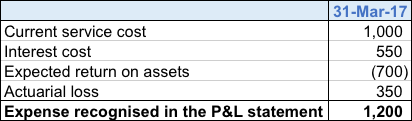Most of the terms in the above table have a specific meaning, though they are not discussed in this post. However, the interpretation of actuarial loss is not intuitive, especially for non-actuarial professionals.

Actuarial gain and loss in the P&L statement is made up of two parts: actuarial loss on the Defined Benefit Obligation (DBO) and actuarial loss on Plan Assets.

### Actuarial gain or loss on DBO

AS 15 reports contain a reconciliation of DBO, such as the table below: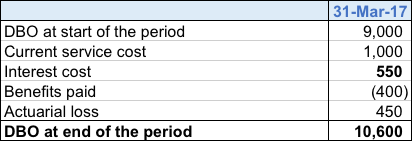The last item; i.e. the DBO at the end of the reporting period is calculated by the actuary using the employee data that the company provides. The actuary also calculates some of the other items in this table, specifically service cost and interest cost. The actuarial loss in this table is the actuarial loss on DBO alone and is the balancing item; to make sure that all the individual line items in the table add up to the last item.

As far as the reconciliation table goes, there is no natural interpretation of actuarial loss, other than being a balancing item. In very broad terms, actuarial loss is the combined impact of everything that didn’t happen ‘as per plan’ during the reporting period.

What could have not gone ‘as per plan’?

An actuarial valuation requires making a number of ‘assumptions’ about future; e.g., discount rate, salary escalation and attrition. Referring to the DBO reconciliation table above, the opening DBO would have made certain assumptions about each of those variables.

A year later, at the time of calculating the closing DBO, the situation could have been somewhat different from the assumptions made at the time when the opening DBO was calculated. For example:

• Discount rate could have changed
• Salary escalation or attrition rate assumption could have changed
• The attrition experience over the reporting period could turn out to be different from what was assumed in the opening DBO
• The salary increments awarded over the reporting period could be different from what was assumed in the opening DBO

If the conditions over the reporting period were exactly the same as the assumptions used in the opening DBO, the actuarial loss would be zero. However, that is unlikely to happen and there will always be some actuarial gain or loss when DBO is calculated at the end of the reporting period.

The first two items are examples of ‘assumption changes’ and the last two are ‘variances’.Actuarial loss on DBO is the sum of all these four impacts. Other sources of actuarial loss could also exist for different types of schemes; e.g. leave availment could be a source of actuarial gain or loss for a compensated absences scheme and post-retirement mortality could be a major source for a pension scheme.

### Actuarial gain or loss on plan assets

Actuarial loss can also arise on the asset side, but here the concept is much simpler compared to the DBO side. Actuarial loss on plan assets is simply the difference between expected return and actual return. This is depicted in the table below: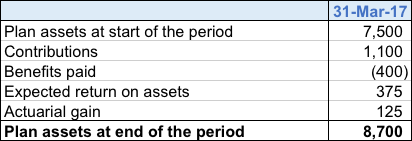### Completing the picture

To summarise, actuarial loss in the P&L statement is the sum of actuarial loss on DBO and actuarial loss on plan assets. It is the combined impact of assumption changes and variances over the reporting period. Companies should try and obtain a detailed breakdown of the actuarial loss as part of their annual statutory valuation, such as the one shown in the table below: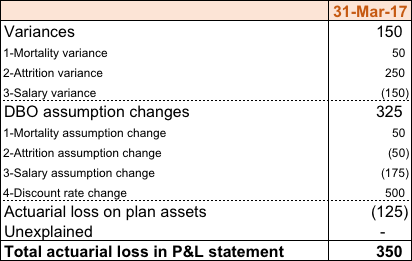Download our guide explaining the basics of actuarial valuation by clicking on the picture below: